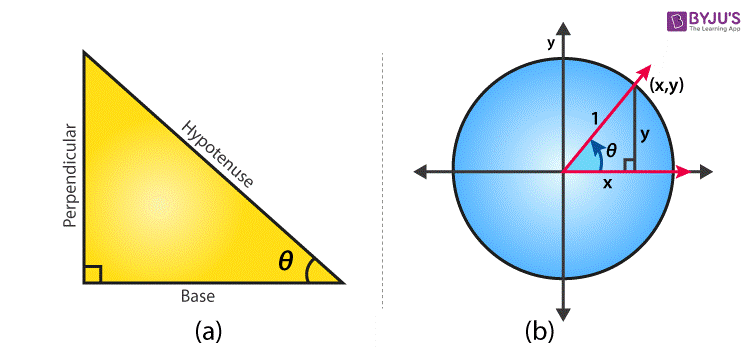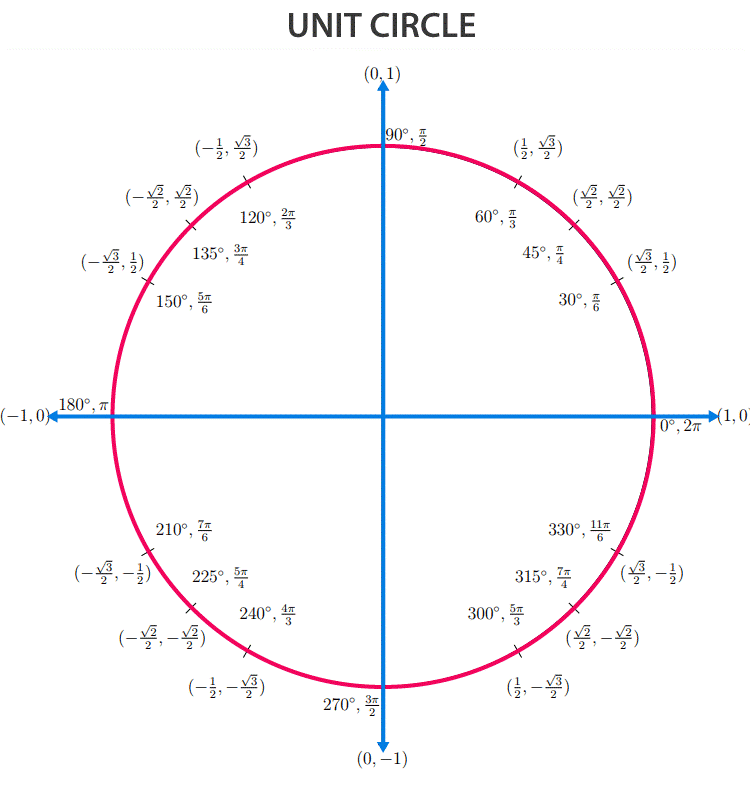Jet Set Go! All about Aeroplanes Jet Set Go! All about Aeroplanes

# Trigonometry Formulas List

Trigonometry formulas list is provided here based on trigonometry ratios such as sine, cosine, tangent, cotangent, secant and cosecant. These formulas are used to solve various trigonometry problems.

In Mathematics, trigonometry is one of the most important topics to learn. Trigonometry is basically the study of triangles where ‘Trigon’ means triangle and ‘metry’ means measurement.

With reference to a right-angled triangle, the list of trigonometry formulas has been formulated. All the trigonometric formulas are based on identities and ratios. The relationship between angles and length of the sides of the triangle is formulated with the help of trigonometry concepts.

The list of trigonometry based formulas will be helpful for students to solve trigonometric problems easily. Below is the list of formulas based on the right-angled triangle and unit circle, which can be used as a reference to study trigonometry.

## List of Important Trigonometry Formulas

First let us learn basic formulas of trigonometry, considering a right-angled triangle, which has an angle θ, a hypotenuse, a side opposite angle to angle θ and a side adjacent to angle θ.### Trigonometric Ratios

So the general trigonometry ratios for a right-angled triangle can be written as;

$$\begin{array}{l}sin\ \theta = \frac{Opposite \, side}{Hypotenuse}\end{array}$$

$$\begin{array}{l}cos\ \theta = \frac{Adjacent \, Side}{Hypotenuse}\end{array}$$

$$\begin{array}{l}tan\ \theta = \frac{Opposite \, side}{Adjacent \, Side}\end{array}$$

$$\begin{array}{l}sec\ \theta = \frac{Hypotenuse}{Adjacent \, side}\end{array}$$

$$\begin{array}{l}cosec\ \theta = \frac{Hypotenuse}{Opposite \, side}\end{array}$$

$$\begin{array}{l}cot\ \theta = \frac{Adjacent \, side}{Opposite \, side}\end{array}$$

### Trigonometric Ratios for Unit Circle

Similarly, for a unit circle, for which the radius is equal to 1, and θ is the angle. The value of the hypotenuse and adjacent side here is equal to the radius of the unit circle.

Hypotenuse = Adjacent side to θ = 1

Therefore, the ratios of trigonometry are given by:

sin θ = y/1 = y

cos θ = x/1 = x

tan θ = y/x

cot θ = x/y

sec θ = 1/x

cosec θ = 1/y

### Trigonometry Identities

Tangent and Cotangent Identities

$$\begin{array}{l}tan\ \theta = \frac{sin\theta }{cos\theta }\end{array}$$

$$\begin{array}{l}cot\ \theta = \frac{cos\theta }{sin\theta }\end{array}$$

Reciprocal Identities

sinθ = 1/cosecθ

cosecθ = 1/sinθ

cosθ = 1/secθ

secθ = 1/cosθ

tanθ = 1/cotθ

cotθ = 1/tanθ

Pythagorean Identities

sin2θ + cos2θ = 1

1 + tan2θ = sec2θ

1 + cot2θ = cosec2θ

### Even and Odd Angle Formulas

sin(-θ) = -sinθ

cos(-θ) = cosθ

tan(-θ) = -tanθ

cot(-θ) = -cotθ

sec(-θ) = secθ

cosec(-θ) = -cosecθ

### Co-function Formulas

sin(900-θ) = cosθ

cos(900-θ) = sinθ

tan(900-θ) = cotθ

cot(900-θ) = tanθ

sec(900-θ) = cosecθ

cosec(900-θ) = secθ

### Double Angle Formulas

sin2θ = 2 sinθ cosθ

cos2θ = 1 – 2sin2θ

$$\begin{array}{l}tan\ 2\theta = \frac{2tan\theta }{1-tan^2\theta }\end{array}$$

### Half Angle Formulas

$$\begin{array}{l}sin\ \theta = \pm \sqrt{\frac{1-cos2\theta }{2}}\end{array}$$

$$\begin{array}{l}cos\ \theta =\pm \sqrt{\frac{1+cos2\theta }{2}}\end{array}$$

$$\begin{array}{l}tan\ \theta = \pm \sqrt{\frac{1-cos2\theta }{1+cos2\theta}}\end{array}$$

### Thrice of Angle Formulas

sin3θ = 3sinθ – 4 sin3θ

Cos 3θ = 4cos3θ – 3 cosθ

$$\begin{array}{l}Tan\ 3\theta = \frac{3 tan\theta – tan^3\theta }{1-3tan^2\theta }\end{array}$$

$$\begin{array}{l}Cot\ 3\theta = \frac{cot^3\theta – 3cot\theta }{3cot^2\theta-1 }\end{array}$$

### Sum and Difference Formulas

Sin (A+B) = Sin A Cos B + Cos A Sin B

Sin (A-B) = Sin A Cos B – Cos A Sin B

Cos (A+B) = Cos A Cos B – Sin A Sin B

Cos (A-B) = Cos A Cos B + Sin A Sin B

$$\begin{array}{l}Tan (A+B) = \frac{Tan A + Tan B}{1 – Tan A Tan B}\end{array}$$

$$\begin{array}{l}Tan (A-B) = \frac{Tan A – Tan B}{1 + Tan A Tan B}\end{array}$$

### Product to Sum Formulas

Sin A Sin B = ½ [Cos (A-B) – Cos (A+B)]

Cos A Cos B = ½ [Cos (A-B) + Cos (A+B)]

Sin A Cos B = ½ [Sin (A+B) + Sin (A-B)]

Cos A Sin B = ½ [Sin (A+B) – Sin (A-B)]

### Sum to Product Formulas

$$\begin{array}{l}Sin A + Sin B = 2 sin \frac{A+B}{2} cos \frac{A-B}{2}\end{array}$$

$$\begin{array}{l}Sin A – Sin B = 2 cos\frac{A+B}{2} sin \frac{A-B}{2}\end{array}$$

$$\begin{array}{l}Cos A + Cos B = 2 cos\frac{A+B}{2} cos\frac{A-B}{2}\end{array}$$

$$\begin{array}{l}Cos A – Cos B = – 2 sin\frac{A+B}{2} sin \frac{A-B}{2}\end{array}$$

### Inverse Trigonometric Functions

If Sin θ = x, then θ = sin-1 x = arcsin(x)

Similarly,

θ = cos-1x = arccos(x)

θ = tan-1 x = arctan(x)

Also, the inverse properties could be defined as;

sin-1(sin θ) = θ

cos-1(cos θ) = θ

tan-1(tan θ) = θ

### Unit Circle

With the help of unit circle, we can see here the different values of sin and cos ratios for different angles such as 0°, 30°, 45°, 60°, 90°, and so on in all the four quadrants.### Trigonometry Table

 Degrees 0° 30° 45° 60° 90° 180° 270° 360° Radians 0 π/6 π/4 π/3 π/2 π 3π/2 2π Sin θ 0 1/2 1/√2 √3/2 1 0 -1 0 Cos θ 1 √3/2 1/√2 1/2 0 -1 0 1 Tan θ 0 1/√3 1 √3 ∞ 0 ∞ 0 Cot θ ∞ √3 1 1/√3 0 ∞ 0 ∞ Sec θ 1 2/√3 √2 2 ∞ -1 ∞ 1 Cosec θ ∞ 2 √2 2/√3 1 ∞ -1 ∞

## Video Lesson on Trigonometry Using FormulasTest your Knowledge on Trigonometry Formulas List

1. PREETHI MAHA

it’s really helpful recall the formulas and it is easy to teach the formulas. thankyou to byjus

2. Balavenkatanathan

THANK YOU GUYS FOR MAKING TRIGONOMETRY EASY ! ! ! ! ! !

3. Its very nice

4. Sourav debnath

Mathematics all formula PDF send me